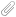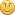September 26, 2022, 07:02:46 AM

## News:

Own IWBasic 2.x ? -----> Get your free upgrade to 3.x now.........

## Matrix Class revisited

Started by REDEBOLT, May 17, 2008, 12:56:18 AM

0 Members and 1 Guest are viewing this topic.

#### REDEBOLT

#####May 17, 2008, 12:56:18 AM
Enclosed for your amusement and edification, I present to you a MATRIX class.  This class exposes methods to create and update matrices, which are two-dimensional arrays.  The matrices are one-based, rather than zero-based as in arrays.  Several methods are available to manipulate matrices.  These methods are illustrated in the file "MATRIX.eba".

`MAT() ' Constructor._MAT() ' Destructor.MATINIT(INT r, INT c) 'Defines this matrix a1()MATCON(DOUBLE value) 'Set all elements of a1() to value of exprMATCONONE() 'Set all elements of a1() to oneMATIDN() 'Establish a1() as an identity matrixMATZER() 'Set all elements of a1() to zeroMATADD(MAT a2, MAT a3) 'Addition a1() = a2() + a3()MATASG(MAT a2) 'Assignment a1() = a2()MATINV(MAT a2) 'Inversion a1() = INV(a2())MATScalarMult(DOUBLE d, MAT a2) 'Scalar Multiply a1() = (expr) * a2()MATSUBT(MAT a2, MAT a3) 'Subtraction a1() = a2() - a3()MATMULT(MAT a2, MAT a3) 'Multiplication a1() = a2() * a3()MATTRN(MAT a2) 'Transposition a1() = TRN(a2()) Accessor functions:GETnr(),INT 'Retrieve number of rows of matrix a1GETnc(),INT 'Retrieve number of columns of matrix a1GetAt(INT row, INT col),DOUBLE 'Retrieve value at row, colSetAt(INT row, INT col, value AS DOUBLE) 'Set value at row, col`

The "MATINV" function code will not be shown since it may be proprietary.  Its functionality is exposed through the "MATINV.DLL/LIB".  This matrix class will become the basis for future discussions on Linear Regression Analysis.

Unzip the "MATRIX.zip" file into a directory of your choice.  The default directory is "MATRIX."  Descriptions of the files follows:

clsMAT.eba   - the class methods
clsMAT.inc   - the class definition.  Copy it to your EBDev/include directory.
EBClean.exe   - used to clean the directory of work files.
MATINV.DLL   - the Matrix Inverse functions.
MATINV.lib   - the library definition.  Copy it to your EBDev/libs directory.
MATRIX.eba   - the source code for the sample program.
MATRIX.exe   - the sample program exe.
WaitKey\$.inc   - the pause routine.  Copy it to your EBDev/include directory.

From the EBasic IDE, create a project with a name of your choosing.  Open the files "clsMAT.eba" and "MATRIX.eba" and insert them into the project.  You are now ready to compile the project.  Press "Build/Rebuild all" or "Ctrl+F5" or click on the "Compile and Execute" button on the toolbar.

Enjoy.Regards,
Bob